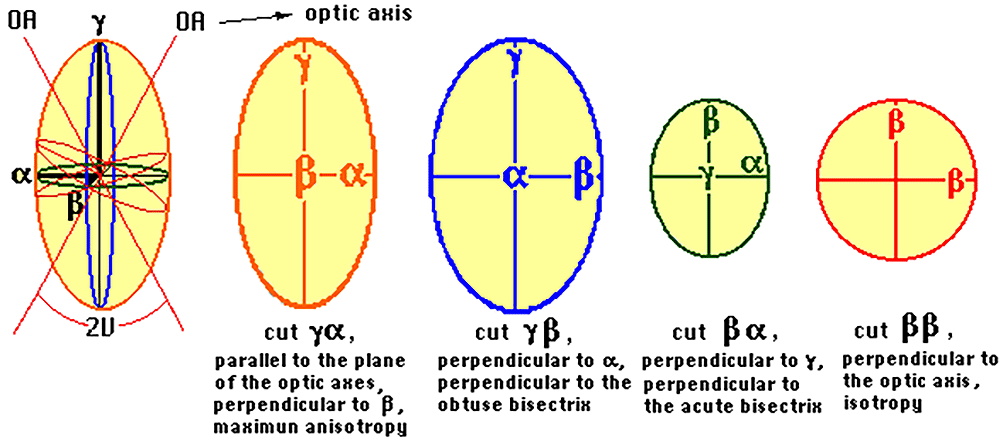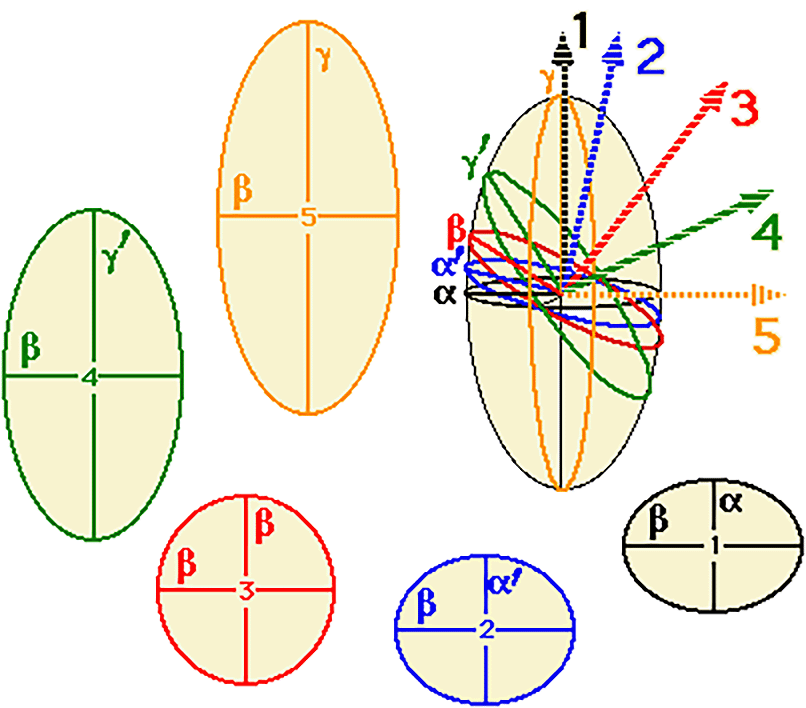Biaxial optical indicatrix

Biaxial optical indicatrix: two optic axes ópticos

In the crystals belonging to the Orthorhombic, Monoclinic and Triclinic systems, the section perpendicular to axis c (vertical) is not the same size, and the equatorial section turns into an ellipse with different axes. The optic indicatrix is an ellipsoid with three axes.

The refractive indices which coincide with the axes of the ellipsoid are known as n alfa, n beta and n gamma, which correspond to the three dimensions (for simplicity the diagram, alfa, beta and gamma has been represented instead of n alfa, n beta and n gamma). It is always true that the smallest refractive index is n alfa, the greatest is n gamma and the intermediate one is n beta, i.e. n alfa < n beta < n gamma.

The indicatrix of these crystals presents two inclined sections which are circular, that is, isotropic.

Perpendicular to each of these sections there is an optic axis. The angle which they form is called the optic angle (2V).Let us consider waves which are propagated in a biaxial crystal according to directions 1,2,3,4 and 5, all within the plane of the diagram. These waves will experience double refraction and the values of their indices will be represented by the intersection of the indicatrix of the plane perpendicular to each ray. These intersections will be ellipses.

For ray 1, with vertical propagation, the directions of vibration of its two waves will move in the horizontal plane. Their refractive indices will correspond to the two main indices n alfa and n beta (the lowest and intermediate).

Ray 2, inclined and thus in the general position, will have refractive indices corresponding to na' and nb. The index n alfa' will have an intermediate value between n alfa and n gamma.

Rays 3,4 and 5 correspond to the ellipses 3,4 and 5.

To go from 1 to 5, we pass through a series of ellipses with one common semi-axis (n beta) and the other semi-axis gradually varying from the lowest index (n alfa) to the highest (n gamma). Therefore, at a certain point of inclination, a section must be reached in which the two semi-axes correspond to nb (remember that this has an intermediate value between n alfa and n gamma) and thus there will be a circumference instead of an ellipse. This will be the case of ray 3, which represents a position of isotropy, i.e. an optic axis.

Symmetrical to this position, but now inclined to the left, another optic axis appears. It is for this reason that these minerals are called biaxial.

For simplicity the diagram, alfa, beta and gamma has been represented instead of n alfa, n beta and n geta.Index | Introduction | Previous | Next | Top